Скачать презентацию Chapter 26 Relativity Copyright The Mc Graw-Hill

991a60ff3901ddd347011f0e42cc7b2c.ppt

• Количество слайдов: 24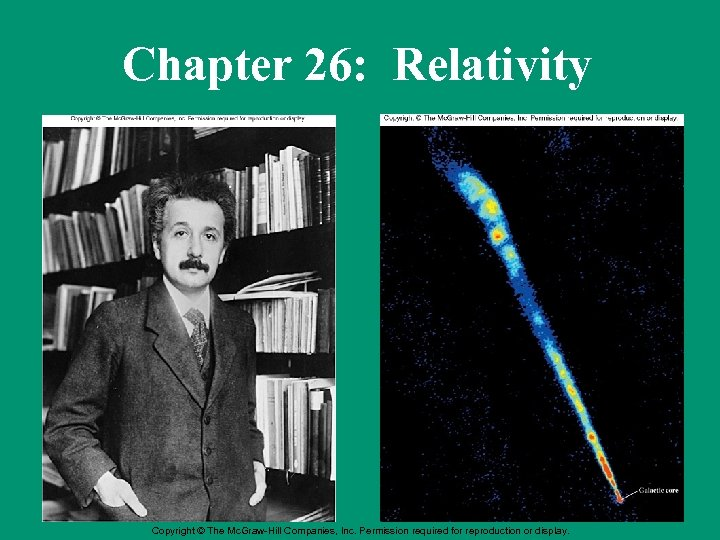Chapter 26: Relativity Copyright © The Mc. Graw-Hill Companies, Inc. Permission required for reproduction or display.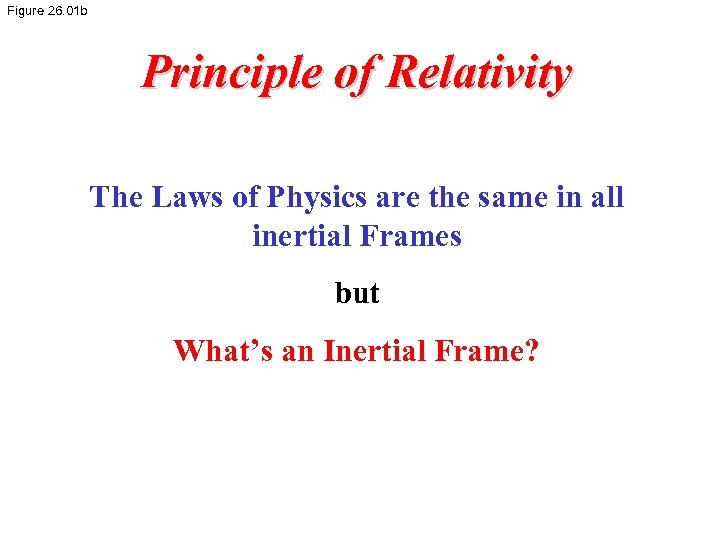Figure 26. 01 b Principle of Relativity The Laws of Physics are the same in all inertial Frames but What’s an Inertial Frame?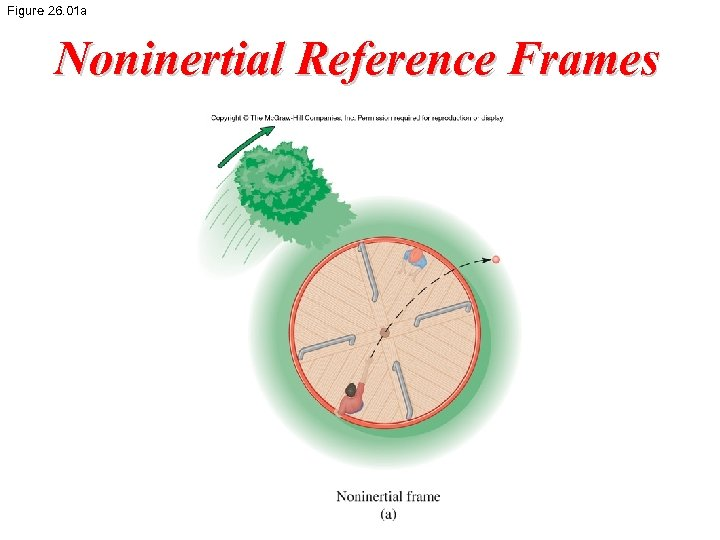Figure 26. 01 a Noninertial Reference Frames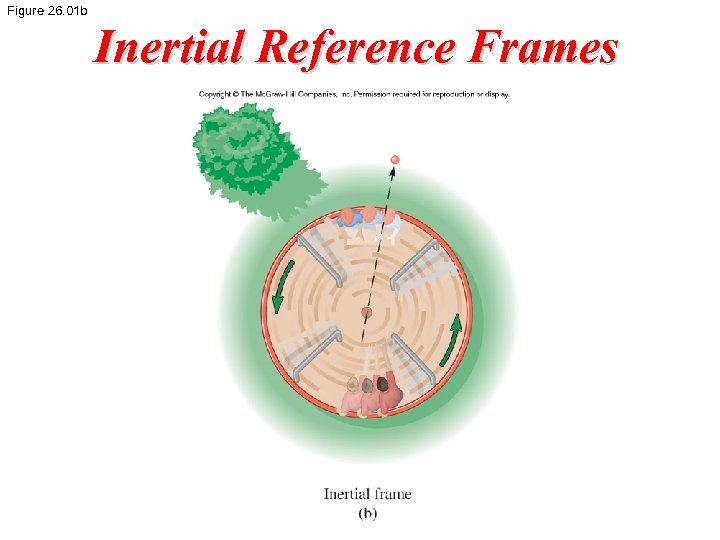Figure 26. 01 b Inertial Reference Frames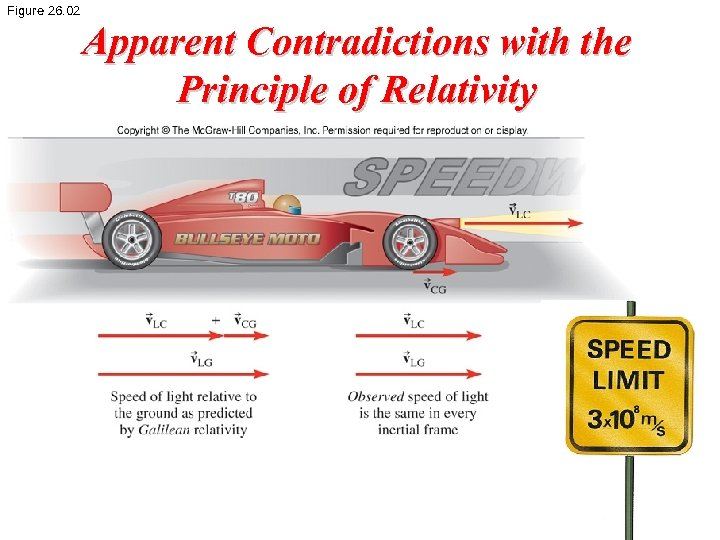Figure 26. 02 Apparent Contradictions with the Principle of Relativity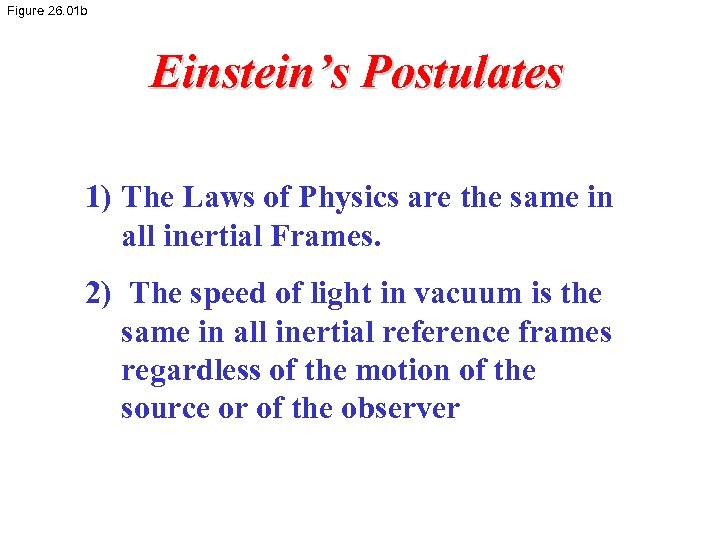Figure 26. 01 b Einstein’s Postulates 1) The Laws of Physics are the same in all inertial Frames. 2) The speed of light in vacuum is the same in all inertial reference frames regardless of the motion of the source or of the observer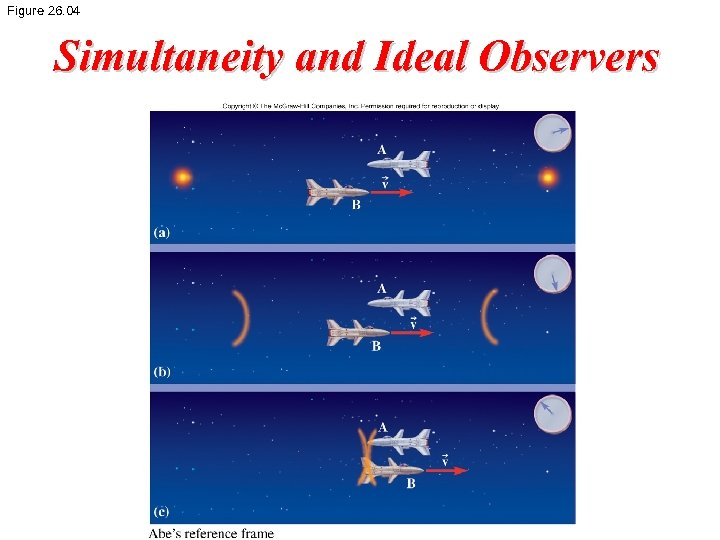Figure 26. 04 Simultaneity and Ideal Observers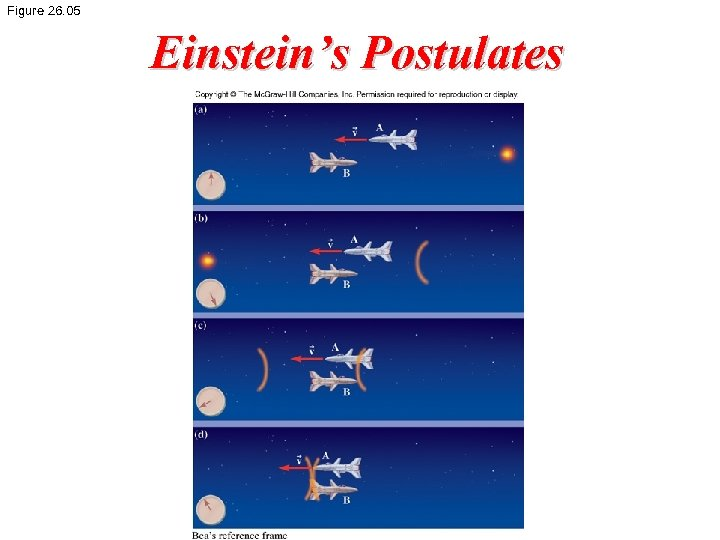Figure 26. 05 Einstein’s Postulates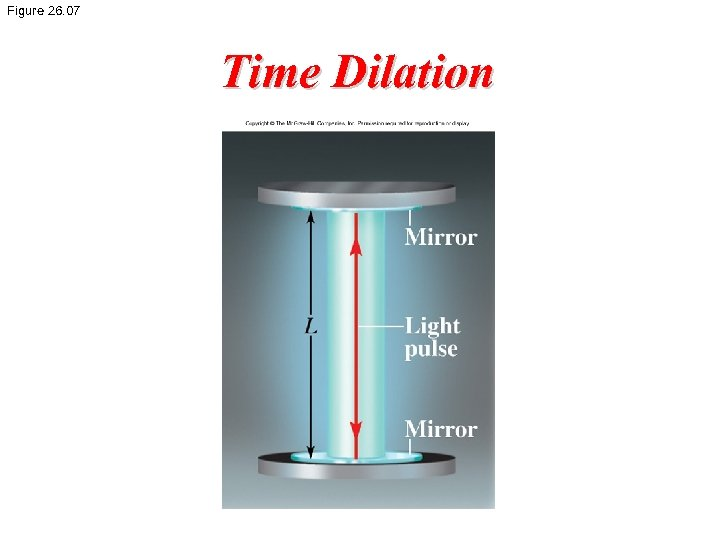Figure 26. 07 Time Dilation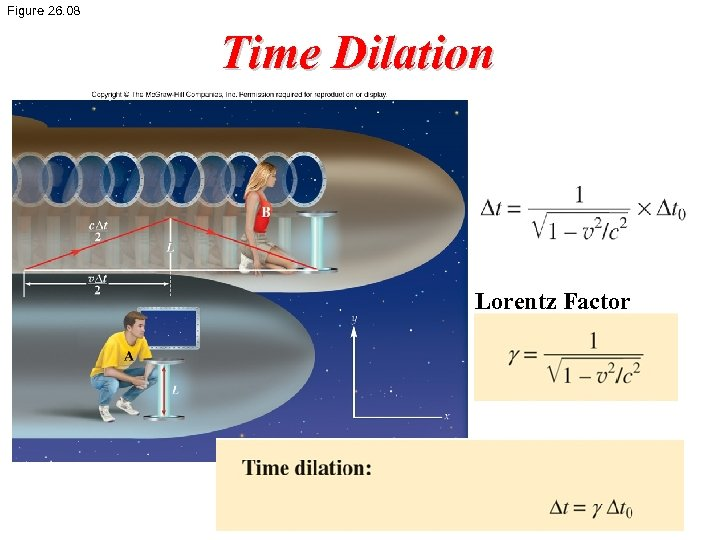Figure 26. 08 Time Dilation Lorentz Factor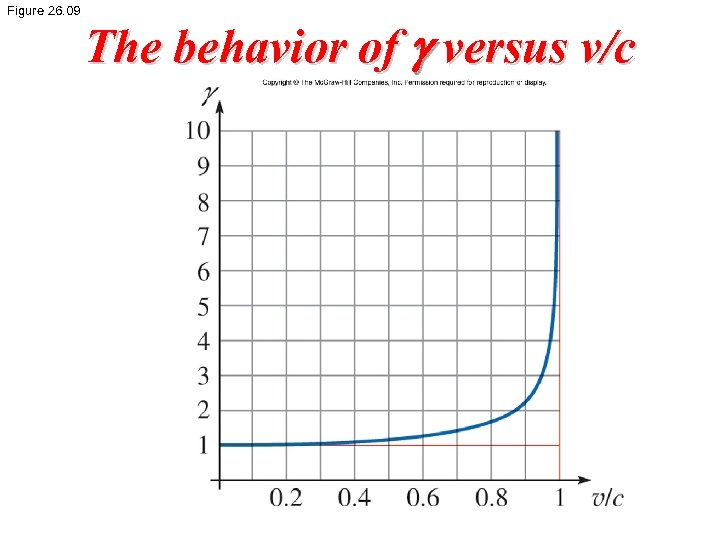Figure 26. 09 The behavior of g versus v/c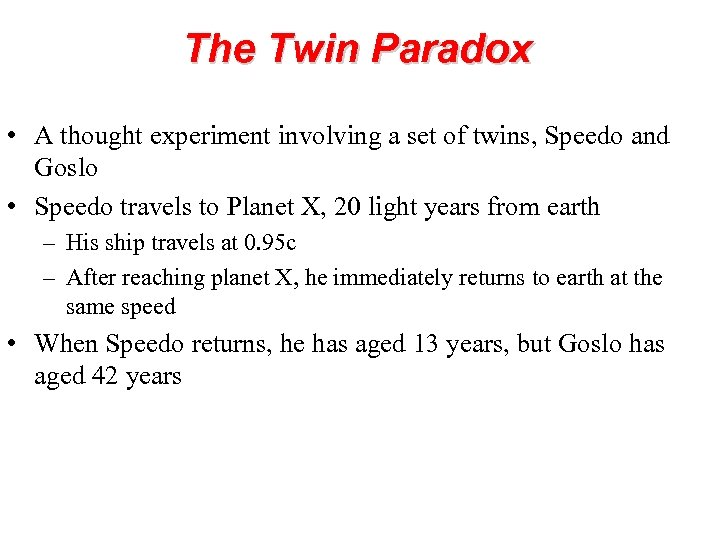The Twin Paradox • A thought experiment involving a set of twins, Speedo and Goslo • Speedo travels to Planet X, 20 light years from earth – His ship travels at 0. 95 c – After reaching planet X, he immediately returns to earth at the same speed • When Speedo returns, he has aged 13 years, but Goslo has aged 42 years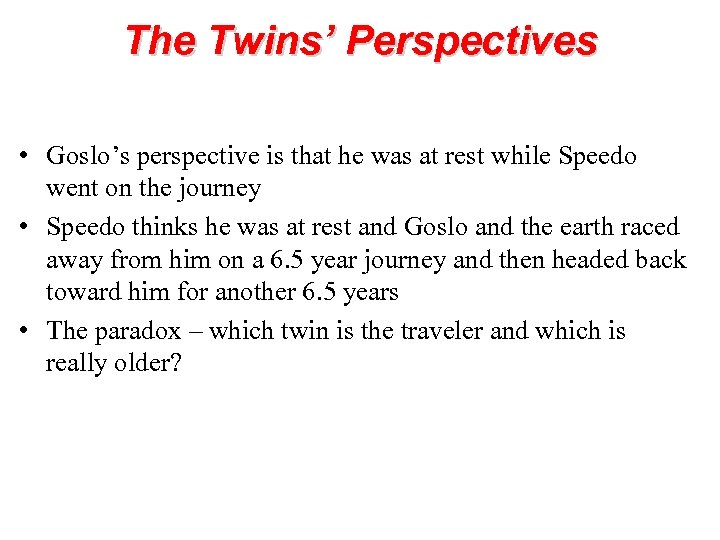The Twins’ Perspectives • Goslo’s perspective is that he was at rest while Speedo went on the journey • Speedo thinks he was at rest and Goslo and the earth raced away from him on a 6. 5 year journey and then headed back toward him for another 6. 5 years • The paradox – which twin is the traveler and which is really older?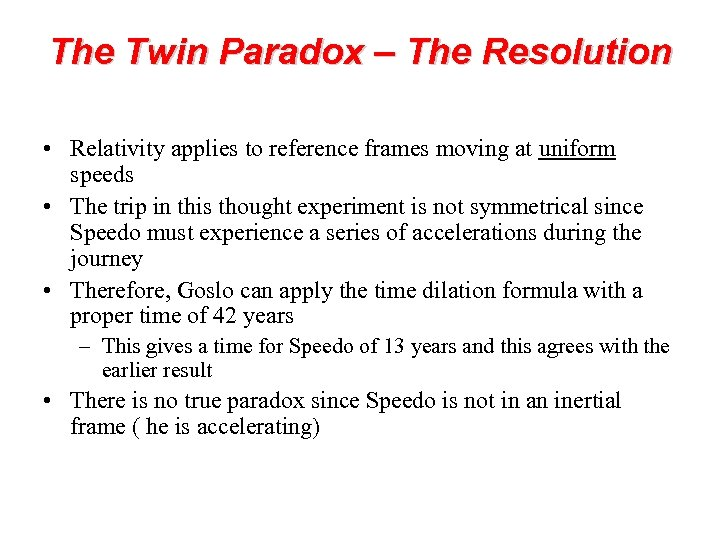The Twin Paradox – The Resolution • Relativity applies to reference frames moving at uniform speeds • The trip in this thought experiment is not symmetrical since Speedo must experience a series of accelerations during the journey • Therefore, Goslo can apply the time dilation formula with a proper time of 42 years – This gives a time for Speedo of 13 years and this agrees with the earlier result • There is no true paradox since Speedo is not in an inertial frame ( he is accelerating)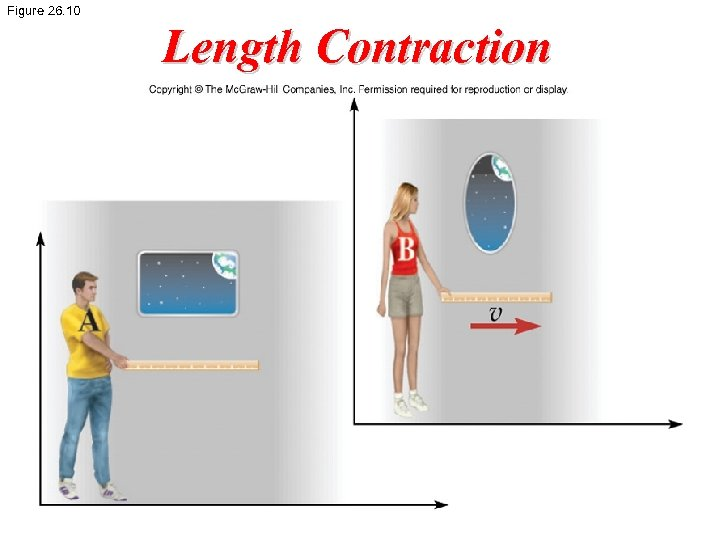Figure 26. 10 Length Contraction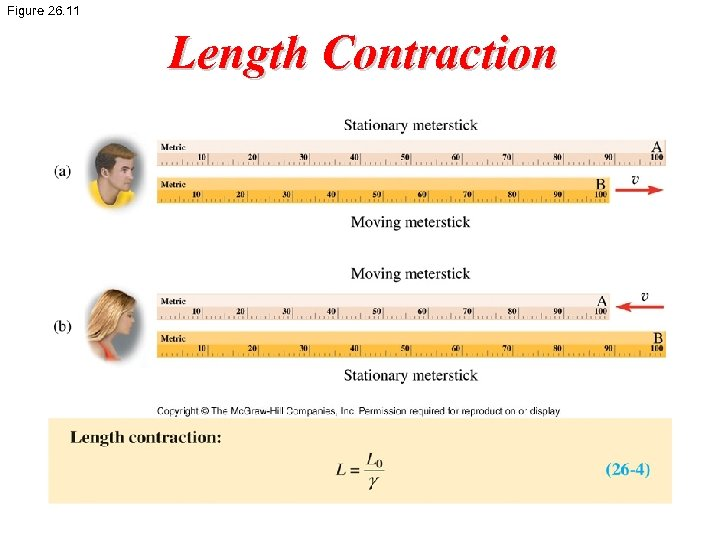Figure 26. 11 Length Contraction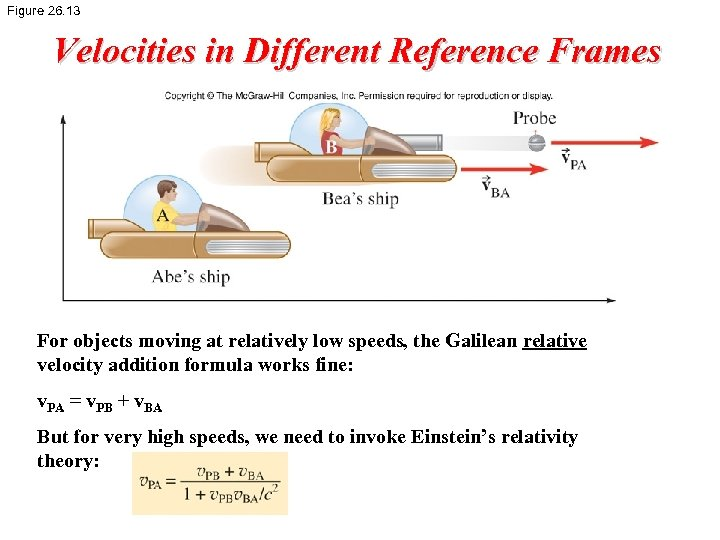Figure 26. 13 Velocities in Different Reference Frames For objects moving at relatively low speeds, the Galilean relative velocity addition formula works fine: v. PA = v. PB + v. BA But for very high speeds, we need to invoke Einstein’s relativity theory: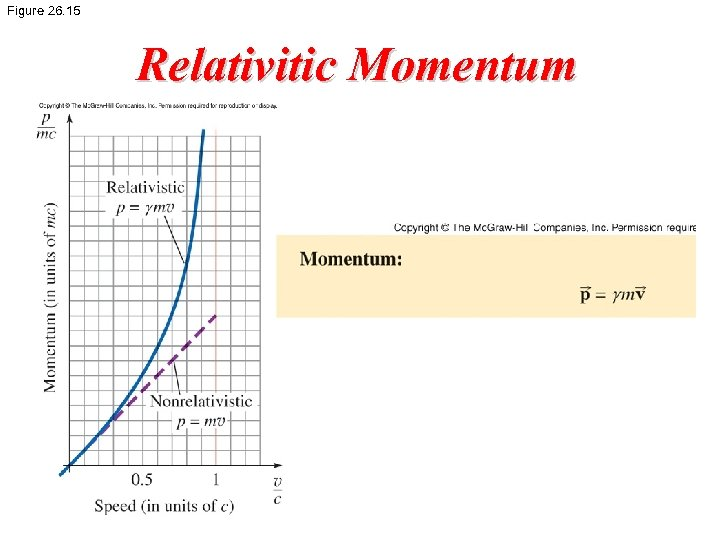Figure 26. 15 Relativitic Momentum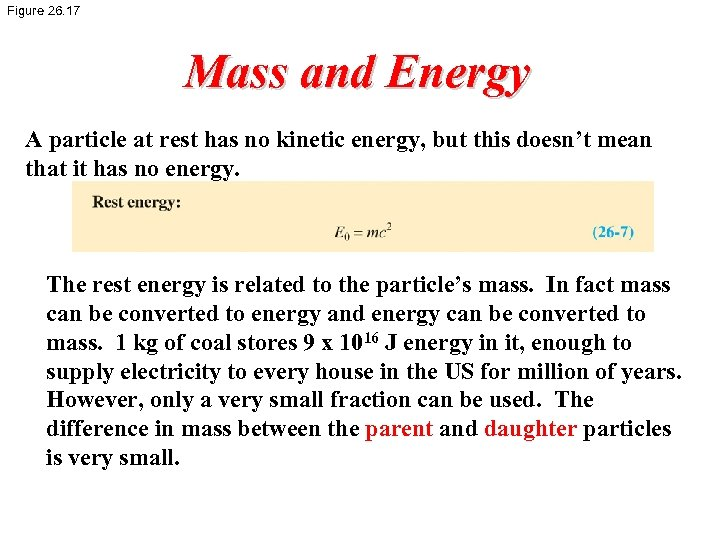Figure 26. 17 Mass and Energy A particle at rest has no kinetic energy, but this doesn’t mean that it has no energy. The rest energy is related to the particle’s mass. In fact mass can be converted to energy and energy can be converted to mass. 1 kg of coal stores 9 x 1016 J energy in it, enough to supply electricity to every house in the US for million of years. However, only a very small fraction can be used. The difference in mass between the parent and daughter particles is very small.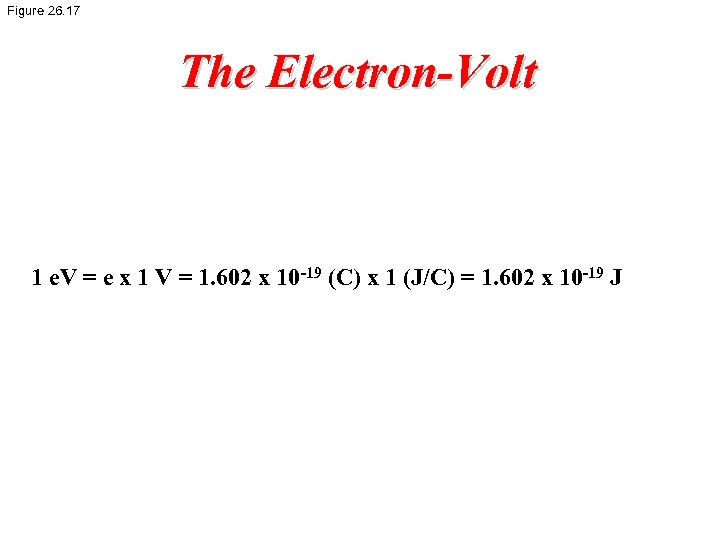Figure 26. 17 The Electron-Volt 1 e. V = e x 1 V = 1. 602 x 10 -19 (C) x 1 (J/C) = 1. 602 x 10 -19 J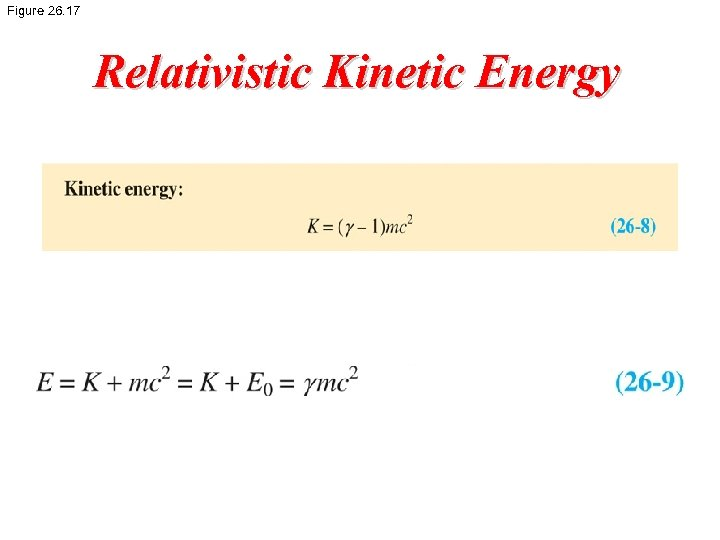Figure 26. 17 Relativistic Kinetic Energy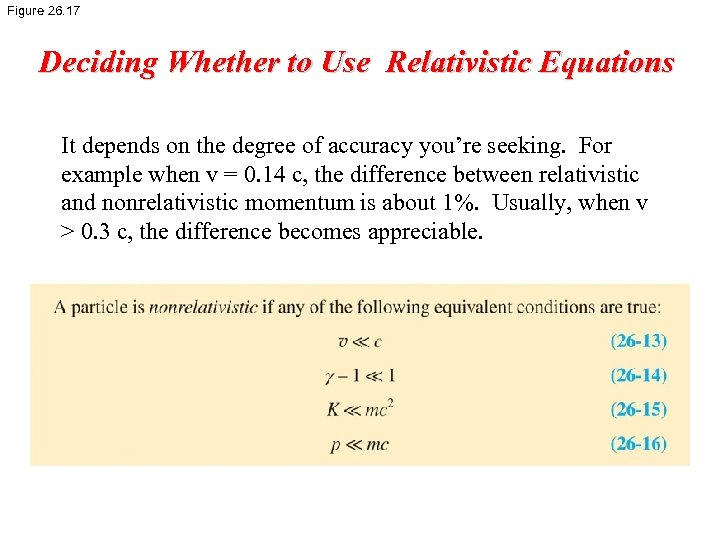Figure 26. 17 Deciding Whether to Use Relativistic Equations It depends on the degree of accuracy you’re seeking. For example when v = 0. 14 c, the difference between relativistic and nonrelativistic momentum is about 1%. Usually, when v > 0. 3 c, the difference becomes appreciable.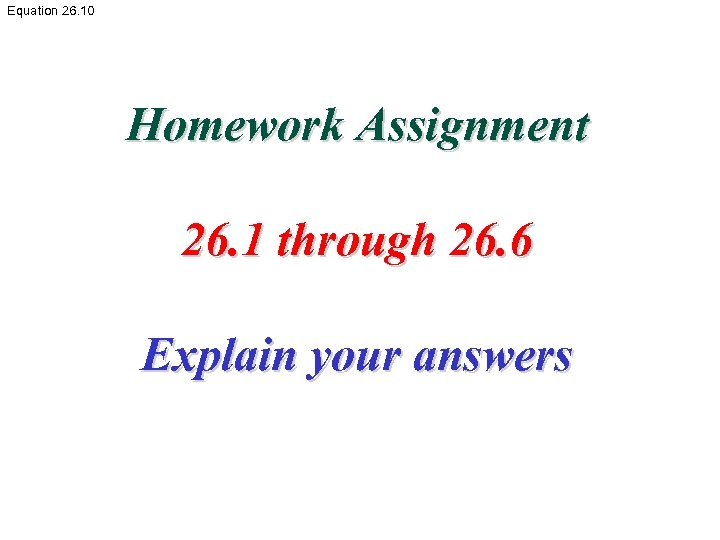Equation 26. 10 Homework Assignment 26. 1 through 26. 6 Explain your answersEquation 26. 11 End of Chapter 26